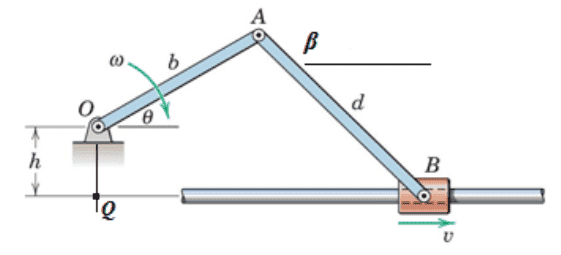# Acceleration of center of mass

## Homework Statement

"Represent (linear) acceleration of center of mass of link OA in terms of variables shown." OmegaOA is given to be some constant value, I have assigned it 'omegaOA'.## Homework Equations

Already derived equations to previous parts of the problem, fairly certain these are correct. Relative variables are neglected, since this is a single body problem. 'a' is linear acceleration, alpha is angular acceleration.
omegaAB = (omegaOA*b*cos(theta))/sqrt(d^2 - (b*sin(theta) + h)^2)
alphaOA = 0
alphaAB = [-(omegaOA^2)*b*sin(theta) - (omegaAB^2)*(b*sin(theta)+h)]/sqrt(d^2 + (b*sin(theta) + h)^2)
alphaOA = (a/r)

## The Attempt at a Solution

If the weight (mg) of the rod was the only force acting on the rod, the problem is very simple.

I*alpha = torque where I = (1/12)*m*length^2
Mass cancels and the problem is easy to solve. However, I am guessing there are force vectors at A that need to be taken into account. Any help would be appreciated.

#### Attachments

haruspex
Homework Helper
Gold Member
2020 Award
If the angular velocity of OA is fixed, and point O is fixed, how does anything to the right of A affect the movement of OA? Are you sure you have stated the question correctly?
And why did you mark the previous thread https://www.physicsforums.com/threads/acceleration-of-center-of-mass.912120/ as solved, then open a new one for the same question?
Sorry about the repost, I had stated the problem poorly the first time, so I figured it would save the time of those replying to make a more precise and accurate post of the question.
And the 'relevant equations' that I have listed are just there because I'm trying to give as much info as possible. And if the reaction forces at A are worked around, there are still support forces at O (according to my analysis, could be wrong). I.e. a simple moment analysis appears to lead to a confusing situation, with either forces at A or O coming up

haruspex
Homework Helper
Gold Member
2020 Award
if the reaction forces at A are worked around,
Why do you care about forces at all? Point O is fixed, the rotation rate of OA is fixed. That tells you everything about the motion of all points on OA.

That makes sense..so the linear acceleration of the center of mass would be zero? I would assume that all points along the link OA would have an acceleration of zero.

haruspex